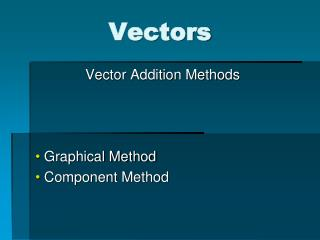DownloadDownload PresentationVectors

# Vectors

Download Presentation## Vectors

- - - - - - - - - - - - - - - - - - - - - - - - - - - E N D - - - - - - - - - - - - - - - - - - - - - - - - - - -
##### Presentation Transcript

1. Vectors Vector Addition Methods Graphical Method Component Method

2. Indicating Direction • For linear (1D) vectors, + and – are used as direction indicators. • For 2-dimensional vectors: • An angle, usually measured from the +x-axis, is reported. • Angles relative to compass directions are also frequently used

3. Drawing Vectors Recall that vector quantities are represented with an arrow, drawn to scale for its magnitude, and in the appropriate direction.

4. Thus, if Vector A is double the magnitude of Vector B will be drawn with an arrow that is twice the length:

5. Vector Addition • Resultant: The term given to the final sum vector when adding 2 or more vectors together.

6. Graphical Vector Addition:Parallelogram Method • Only useful only for adding 2 vectors • Vectors are commutative • It doesn’t matter how you add them, the answer is the same!

7. Graphical Vector Addition: Tip-to-Tail • Step 1: Carefully draw, to scale, the first vector. • Step 2: At the tip of the first vector, as if you are continuing a path, start drawing the second vector. • S tep 3: Draw the resultant vector from the tail of Vector 1 to the tip of Vector 2 (start to finish).

8. Sample Problem:What’s the Vector, Victor? • Graphically add the following vectors and find the resultant displacement including direction • 50 meters (m) @ 45º S of E • 70 meters (m) @ 30º S of W

9. Vector Components • Every vector can be “broken down” into its x- and y-components • Components: the horizontal and vertical legs of a right triangle • The original vector is the hypotenuse of the right triangle!

10. Calculating Vector Components

11. Component Method of Vector Addition Step 1: Resolve all individual vectors into their x- and y- components, remembering to indicate a positive or negative direction appropriately

12. Component Method of Vector Addition: Step 2: Determine the sum of each of the x-components This sum is equal to the magnitude (and direction) of the resultant’s x-component

13. Component Method of Vector Addition: Step 3: Determine the sum of each of the y-components This sum is equal to the magnitude (and direction) of the resultant’s y-component

14. Vector Diagrams

15. Component Method of Vector Addition: • Step 4: Use the Pythagorean Theorem to determine the magnitude of the final resultant vector: • In a general format: • R = resultant vector’s magnitude • Rx = resultant x-component (sum of all x-components) • Ry = resultant y-component (sum of all y-components)

16. Component Method of Vector Addition: Step 5: Use trigonometry to find the resultant vector’s direction:

17. Sample Problem:As the Parakeet Flies?! • Pat and his pet parakeet Pete are at the Pike’s Place Market downtown purchasing papayas. They decide to race to the library that is 3 blocks East and 5 blocks South. • Draw a scaled drawing showing Pat’s path along the streets and Pete’s path as the parakeet flies (straight to the destination). • How far does Pete have to fly? In what direction?